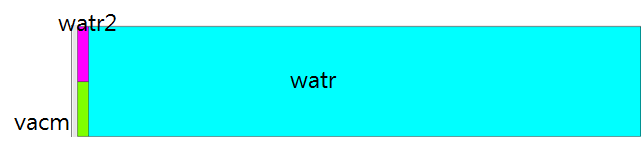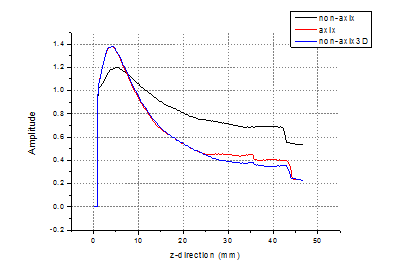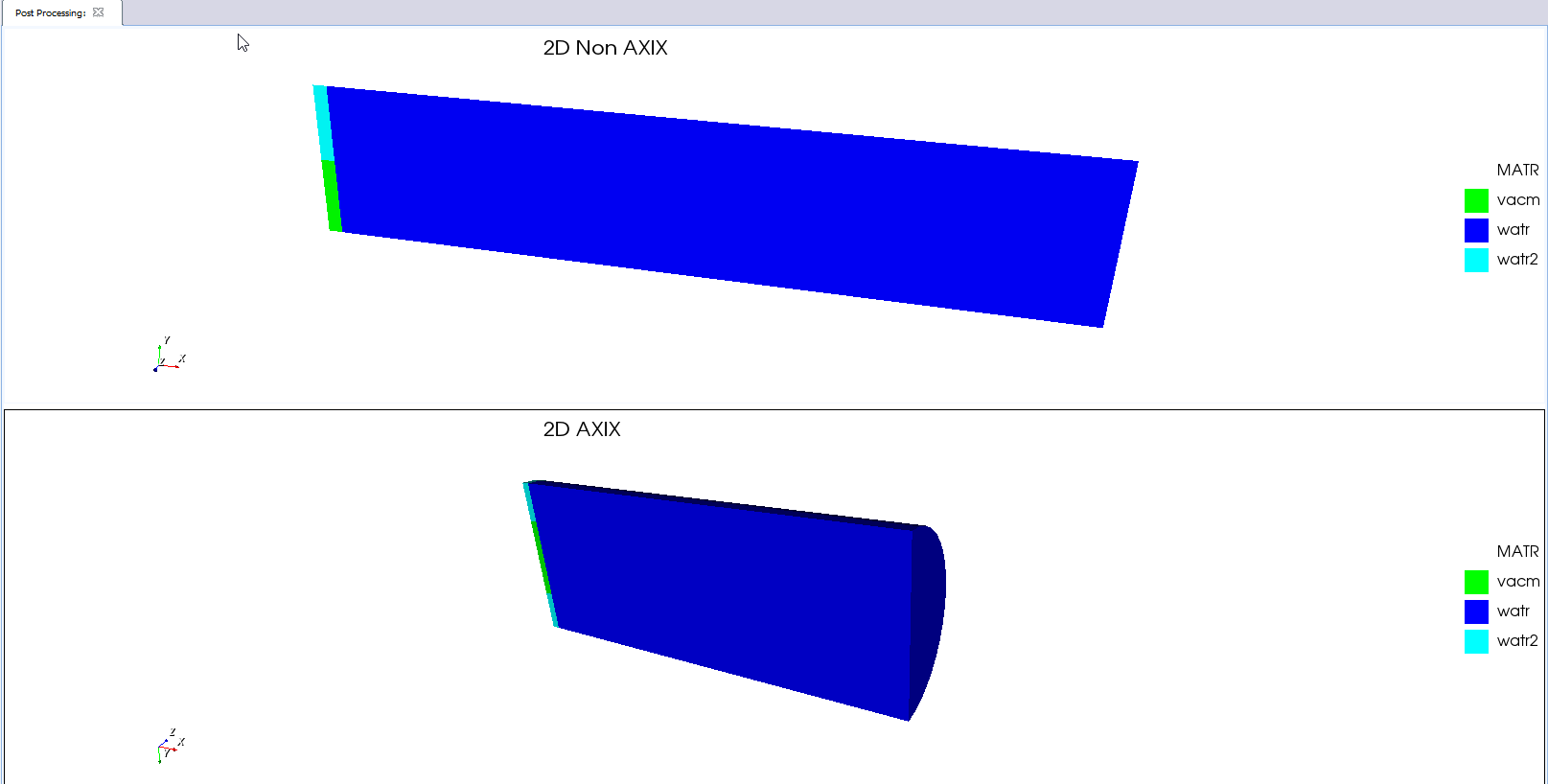# How can I understand about different result between axix and non-axix? Answered

Hi.

I need to understand about different result between axix and non-axix before I use result.

I designed simple piston source and calculate maximum and minimum pressure field data.

There are two case.

1. grid \$indgrd \$jndgrd axix (using axisymmetric)

2. grid \$indgrd \$jndgrd (just plain strain)

This is my modeling figure. Simple.Continuous sine wave comes out from vacm foward watr (linear).

Every code are same, except grid part. But result is quite different.

This graph plot maximum field data along with center line. (y=0)We have same result from axix and 3D. but not non-axix.

Why?

Every situation is not axi-symmetric.

I think non-axix and axix result must be same or close same.

This prove step is very important to me because I have many cases not axi-symmetric such as skull.

------------------------------------------

symb x1 = 0.0
symb x2 = \$x1 + 1e-3
symb x3 = \$x2 + 50e-3

symb y1 = 0.0
symb y2 = \$y1 + 5e-3
symb y3 = \$y2 + 5e-3

symb freqint = 0.2e6
symb wavevel = 1500
symb wavemin = \$wavevel / \$freqint
symb nelem = 30
symb box = \$wavemin / \$nelem

symb i1 = 1
symb i2 = \$i1 + nint ( ( \$x2 - \$x1 ) / \$box )
symb i3 = \$i2 + nint ( ( \$x3 - \$x2 ) / \$box )
symb indgrd = \$i3

symb j1 = 1
symb j2 = \$j1 + nint ( ( \$y2 - \$y1 ) / \$box )
symb j3 = \$j2 + nint ( ( \$y3 - \$y2 ) / \$box )
symb jndgrd = \$j3

grid \$indgrd \$jndgrd axix

geom
keypnt 3 3
end

symb epvacm = 8.854e-12 /* dielectric constant for vacumn
symb freqdamp = 1.e6 if noexist /* specified frequency for damping model
symb rmu0 = 1.2566e-6

matr
type elas
wvsp on
prop watr 1000. 1496. 0. 0.01 0.0 0.0
symb eps = \$epvacm * 1.0
elec watr \$eps
copy watr watr2

wvsp on
type elas
prop vacm 0 0 0
symb eps = 1
symb aeps = \$epvacm * \$eps
elec vacm \$aeps
end

site
regn watr2
regn vacm \$i1 \$i2 \$j1 \$j2
regn watr \$i2 \$i3 \$j1 \$j3
end

grph
plot matr
end
term

boun
side xmin absr stnd
side xmax absr stnd
side ymin symm all
side ymax absr stnd
end

func sine 200e3 1. 0. 0.

calc
pres aprs
max aprs pmin pmax
end

plod
pdef pld1 func /* choose driving function and name 'pld1'
vctr vct1 1. 0. 0.
sdef pld1 vct1 \$i2 \$i2 \$j1 \$j2
end

pout
hist func
end

prcs /* run process step

symb #get { step } timestep
symb simtime = \$x3 / \$wavevel
symb nexec = nint ( \$simtime / \$step )
symb nloops = 50
symb nexec_loop = nint ( \$nexec / \$nloops )

grph
set imag avi /* Movie generation
end

proc plot save

exec \$nexec_loop

grph
nvew 4 1
colr tabl data 6 /* Set color table for data
mirr y
plot pmax
plot aprs
plot pmin
imag /* Save frame of movie - creates AVI in folder when model executes fully
end
end\$ proc

proc plot \$nloops

stop

• Hi Hyunjae Song,

Well the axix model is revolved around the x-axis creating a cylindrical model where the radius of the model is determined by the length along the y-axis.

https://support.onscale.com/hc/en-us/articles/360002113278-Creating-a-New-Project

If you created a cylindrical 3D model you would find it will match up with the 2D axix model.

Hope this helps

Regards,

Oliver

• Hi. Oliver.

But I know there are same result 3D and 2D axix model.

I post graph that show 3D, 2D axix model result is same but not 2D plain.

I think even 2D plain stain, it match with 2D axix model.

Because every subject is not axi-symmetric like skull.

My final goal is simulate using skull MRI image.

But this image is 2D, not axi-symmetric model.

How can I sure using 2D simulation result is right?

If 2D and 2D axix model has same result, I can tell simulation result is correct.

But before then, I can't tell it is right.

#### This mismatch between 2D and 2D axix result happen at onscale too.

• Sorry but I don't understand what you are trying to do or compare here...

Yes you are seeing a close match in results between 2D-AXIX and the 3D model because these models will be exactly the same.. the 2D PLANE STRAIN model doesn't have a depth, see below:If you have a model/subject such as a skull that can't be approximated with a 2D-AXIX, AXIY or any other symettry conditions then the likely course is to model it in full 2D or 3D without any of these conditions.

I understand you're concerns and your desire to verify the results but you simply can't and won't get the results of 2D PLANE STRAIN model to match with any of the 2D AXIX or AXIY models.

What we usually suggest is users begin with either a 2D PLAIN STRAIN or 2D AXI model get results and verify them with theory or real life simulation data then move to 3D.

I hope this is clear.

Oliver

• Thank you. Oliver.

Yes. I agree what you mentioned about step, 2D plain stain or 2D axi model verify first, and then move to 3D.

How can I verity my 2D plain stain result is right?

Do I have to solve wave equation using other programs?

Is there a way to solve or verify in onscale?

• Hi Hyunjae,

You can verify the 2D PLAIN STRAIN against a 3D model similar to what you have done with the 2D AXI and 3D model. What I'm trying to say is using the same 3D model to verify both 2D cases will never work because the geometries are completely different.

The 3D model in this case shouldn't be cylindrical in shape.

If you're models are all cylindrical in shape then tbh I would suggest moving away from the PLAIN STRAIN model... Do you have any physical results measured in a lab or similar that you can compare the simulation results with?